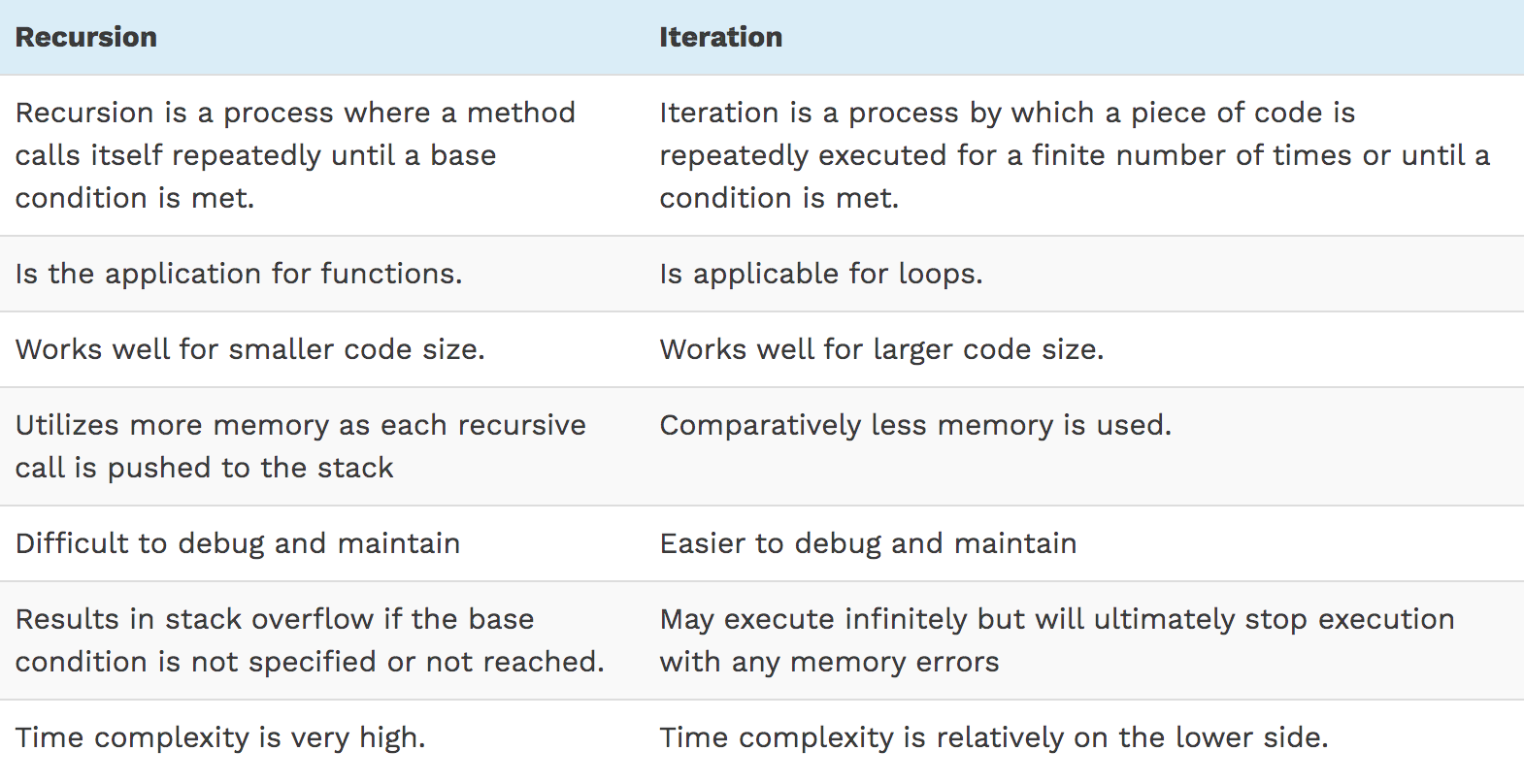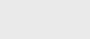3年前 (2020-10-03) |   抢沙发  909

## Java中的递归是什么？

### 递归语法

1. 可以调用自身的方法调用，即递归调用
2. 停止递归的先决条件。

``````methodName (T parameters…)
{
if (precondition == true)

//precondition or base condition
{
return result;
}
return methodName (T parameters…);

//recursive call
}
``````

## 理解Java中的递归

### 递归基础条件

``````public class Main{
static int fact(int n)
{
if (n == 1)

// base condition
return 1;
else
return n*fact(n-1);
}    public static void main(String[] args) {
int result = fact(10);

System.out.println("10! = " + result);
}
}``````

``10 != 3628800``

### 递归时出现堆栈溢出错误

``````public class Main
{
static int fact(int n)
{
if (n == 100)

// base condition resulting in stack overflow
return 1;
else
return n*fact(n-1);
}
public static void main(String[] args) {
int result = fact(10);

System.out.println("10! = " + result);
}
}``````

## Java中的递归示例

### 使用递归的Fibonacci级数

1,1,2,3,5,8,13,21,34,55，…

``````public class Main
{
//method to calculate fibonacci series
static int fibonacci(int n) {
if (n <= 1) {
return n;
}
return fibonacci(n-1) + fibonacci(n-2);
}
public static void main(String[] args) {
int number = 10;

//print first 10 numbers of fibonacci series
System.out.println ("Fibonacci Series: First 10 numbers:");
for (int i = 1; i <= number; i++)
{
System.out.print(fibonacci(i) + " ");
}

}
}
``````

``````Fibonacci Series: First 10 numbers:

1 1 2 3 5 8 13 21 34 55``````

### 使用递归检查数字是否为回文Palindrome

``````import java.io.*;
import java.util.*;

public class Main {
// check if num contains only one digit
public static int oneDigit(int num) {
if ((num >= 0) && (num < 10))
return 1;
else
return 0;
}
//palindrome utility function
public static int isPalindrome_util (int num, int revNum) throws Exception {
// base condition; return if num=0
if (num == 0) {
return revNum;
} else { //call utility function recursively
revNum = isPalindrome_util(num / 10, revNum);
}
// Check if first digit of num and revNum are equal
if (num % 10 == revNum % 10) {
// if yes, revNum will move with num
return revNum / 10;
}
else {
// exit
throw new Exception();
}
}
//method to check if a given number is palindrome using palindrome utility function
public static int isPalindrome(int num)  throws Exception {
if (num < 0)
num = (-num);

int revNum = (num);
return isPalindrome_util(num, revNum);
}

public static void main(String args[]) {
int n = 1242;
try {
isPalindrome(n);
System.out.println("Yes, the given number " + n + " is a palindrome.");
} catch (Exception e) {
System.out.println("No, the given number " + n + " is not a palindrome.");
}
n = 1221;
try {
isPalindrome(n);
System.out.println("Yes, the given number " + n + " is a palindrome.");
} catch (Exception e) {
System.out.println("No, the given number " + n + " is not a palindrome.");
}
}
}``````

``````No, the given number 1242 is not a palindrome.

Yes, the given number 1221 is a palindrome.``````

### 反向字符串递归Java

``````class String_Reverse
{
//recursive method to reverse a given string
void reverseString(String str)
{
//base condition; return if string is null or with 1 or less character
if ((str==null)||(str.length() <= 1))
System.out.println(str);
else
{
//recursively print each character in the string from the end
System.out.print(str.charAt(str.length()-1));
reverseString(str.substring(0,str.length()-1));
}
}
}
class Main{
public static void main(String[] args)
{
String inputstr = "SoftwareTestingHelp";
System.out.println("The given string: " + inputstr);
String_Reverse obj = new String_Reverse();
System.out.print("The reversed string: ");
obj.reverseString(inputstr);

}
}
``````

``````The given string: SoftwareTestingHelp

The reversed string: pleHgnitseTerawtfoS``````

### 二进制搜索Java递归

``````import java.util.*;
class Binary_Search {
// recursive binary search
int binarySearch(int numArray[], int left, int right, int key)   {
if (right >= left) {
//calculate mid of the array
int mid = left + (right - left) / 2;
// if the key is at mid, return mid
if (numArray[mid] == key)
return mid;
// if key < mid, recursively search the left subarray
if (numArray[mid] > key)
return binarySearch(numArray, left, mid - 1, key);
// Else recursively search in the right subarray
return binarySearch(numArray, mid + 1, right, key);
}
// no elements in the array, return -1
return -1;
}
}
class Main{
public static void main(String args[])   {
Binary_Search ob = new Binary_Search();
//declare and print the array
int numArray[] = { 4,6,12,16,22};
System.out.println("The given array : " + Arrays.toString(numArray));
int len = numArray.length;              //length of the array
int key = 16;                           //key to be searched
int result = ob.binarySearch(numArray, 0, len - 1, key);
if (result == -1)
System.out.println("Element " + key + " not present");
else
System.out.println("Element " + key + " found at index " + result);
}
}``````

``````The given array : [4, 6, 12, 16, 22]

Element 16 found at index 3``````

### 使用递归查找数组中的最小值

``````import java.util.*;
class Main
{
static int getMin(int numArray[], int i, int n)
{
//return first element if only one element or minimum of the array elements
return (n == 1) ? numArray[i] :
Math.min(numArray[i], getMin(numArray,i + 1 , n - 1));
}

public static void main(String[] args)
{
int numArray[] = { 7,32,64,2,10,23 };
System.out.println("Given Array : " + Arrays.toString(numArray));
int n = numArray.length;
System.out.print("Minimum element of array: " + getMin(numArray, 0, n) + "\n");
}
}
``````

``````Given Array : [7, 32, 64, 2, 10, 23]

Minimum element of array: 2``````

## 递归类型

### 尾递归

``````methodName ( T parameters…){
{
if (base_condition == true)
{
return result;
}
return methodName (T parameters …)      //tail recursion
}``````

### 头递归

``````methodName (T parameters…){
if (some_condition == true)
{
return methodName (T parameters…);
}
return result;
}``````

## Java中的递归与迭代区别:## 总结### 注册